### Home > CALC > Chapter Ch2 > Lesson 2.4.1 > Problem2-145

2-145.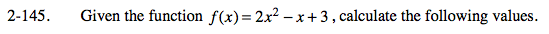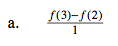This is the slope of the secant between x = 3 and x = 2, otherwise known as Average Rate of Change (AROC).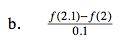This is the slope of the secant between x = 2.1 and x = 2.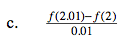And this is the slope of the secant between x =____ and x =____.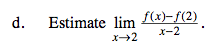Notice that as you progressed through parts (a), (b) and (c), the secant line becomes smaller and smaller, squeezing in on the actual slope at x = 2. Based on the patterns you see as the secant line decreases, what do you think the slope of the smallest possible secant line will be?